# Inverse Operations: Multiplication and Division

Inverse Operations: Multiplication and Division#### Writing Multiplication as Division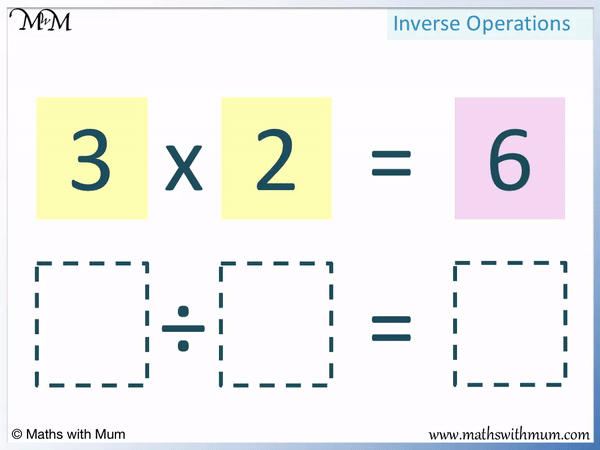• Multiplication and division are inverse operations. This means that they are opposites.
• All multiplication sentences can be rewritten as division sentences and all division sentences can be rewritten as multiplication sentences.
• Every multiplication sentence can be written as two different division sentences.
• Here is the multiplication sentence 3 × 2 = 6.
• The answer of a multiplication sentence is the number that is divided at the beginning of a division sentence.
• The answer of 6 is written at the start of the division sentence.
• The two numbers that are multiplied together are then written next in the division sentence.
• 3 × 2 = 6 can be written as 6 ÷ 3 = 2 or 6 ÷ 2 = 3.
• Two numbers are multiplied together in the multiplication sentence. One is the number we divide by in the division sentence and the other is the answer.

#### Writing Division as Multiplication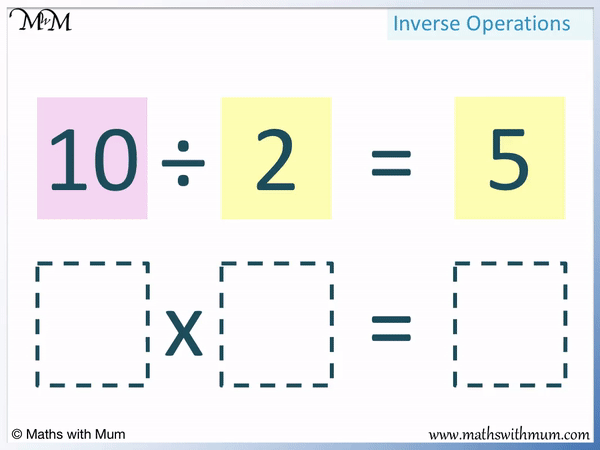• To write a division sentence as a multiplication sentence, simply rearrange the numbers.
• Every division sentence can be written as two different multiplication sentences.
• The number that is being divided in the division sentence is now the answer of the multiplication sentence.
• 10 is being divided and so, it is the answer of the multiplication sentence.
• The number being divided by and the answer of a division sentence are both written multiplied together.
• The answer of 6 is written at the start of the division sentence.
• The two numbers that are multiplied together are then written next in the division sentence.
• 10 ÷ 2 = 5 can be written as 2 × 5 = 10 or 5 × 2 = 10.

Multiplication and division are opposites.

Division and multiplication sentences can be made by rearranging the numbers.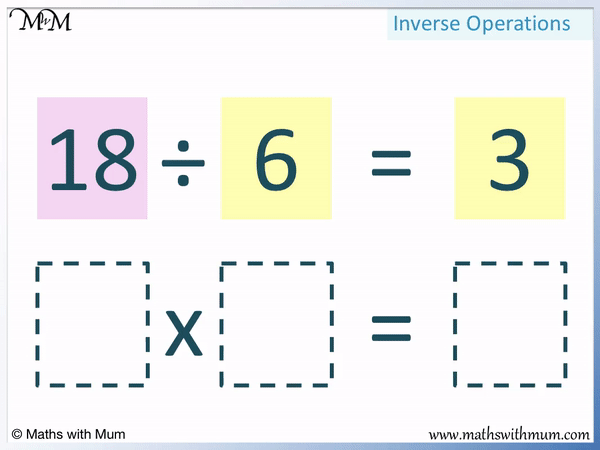• We can write every division sentence as a multiplication sentence.
• The number being divided by is the answer to a multiplication sentence.
• 18 moves from the start of the division to become the answer of the multiplication.
• The number being divided by and the answer to the division are both multiplied together in the multiplication sentence.
• 18 ÷ 6 = 3 can be written as 3 × 6 = 18 or 6 × 3 = 18.#### Writing Division as Multiplication# Inverse Operations: Multiplication and Division

## What are Inverse Operations?

Inverse operations are two different types of calculation that have the opposite effect on each other. For example, subtraction is the inverse operation to addition and division is the inverse operation to multiplication.

In maths, the word inverse means the opposite.

Inverse operations can be used to undo the original operation. This has the effect of returning a value that we started with before the first calculation.

For example, we can start with the number 5 and then multiply it by 4.

5 × 4 = 20. We started with 5 but now have 20.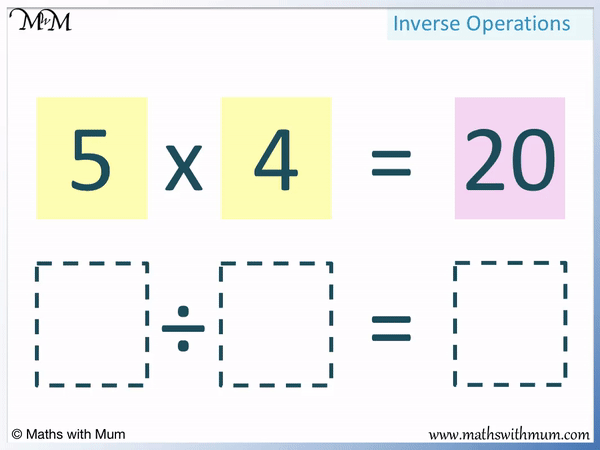To return from 20 to 5, we use the inverse operation.

20 ÷ 4 = 5. We can return from 20 to 5 by dividing by 4.

We say that multiplying by 4 is the inverse operation to dividing by 4.

Multiplying 5 by 4 resulted in 20.

Dividing 20 by 4 took us back to 5.

Multiplication and division are examples of inverse operations.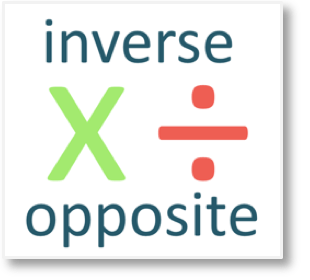## What is the Inverse of Multiplication?

The inverse of multiplication is division. If you multiply by a given number and then divide by the same number, you will arrive at the same number you started with. Division has the opposite effect to multiplication.

For example here is 3 × 2 = 6.

We started with the number 3.

We multiplied it by 2 to increase 3 to 6.An inverse operation must be used to return from 6 to 3.

The opposite of multiplying by 2 is to divide by 2.

We divide 6 by 2 to return to our original number of 3.

Division had the inverse effect to the multiplication done in the original calculation.

Division and multiplication are only inverses if the division and multiplication are by the same number. In this example we multiplied and divided by 2.

## What is the Inverse of Division?

The inverse of division is multiplication. If you divide by a given number and then multiply by the same number, you will arrive at the same number you started with. Multiplication has the opposite effect to division.

For example, here is 10 ÷ 2 = 5.

We started with the number 10.

We divided it by 2 to decrease 10 to 5.To return from 5 to our original number of 10, we must use an inverse operation.

We can multiply 5 by 2 to get back to our original number of 10. 5 × 2 = 10.

Dividing by 2 and multiplying by 2 are inverse operations. Multiplying by 2 had the effect of undoing the division by 2.

## How to Write Multiplication as Division

To write a multiplication as a division, follow these steps:

1. Write the answer of the multiplication at the start of the division before the division sign.
2. Write the two numbers being multiplied after the division sign and after the equals sign respectively.
3. The two numbers in step two can be written in either order to form two different divison sentences.

For example, here is 7 × 8 = 56. We will write this multiplication as a division.

Every multiplication can be rewritten as a division using the same numbers but in a different order.

If you know the answer to a given multiplication, you can immediately use this to create a division without performing any calculations. Simply rearrange the numbers.

The first step is to write the answer to the multiplication at the start of the division sentence. The answer of the multiplication is now the number being divided by.

56 is the answer to the multiplication in 7 × 8 = 56. We will start our division with 56.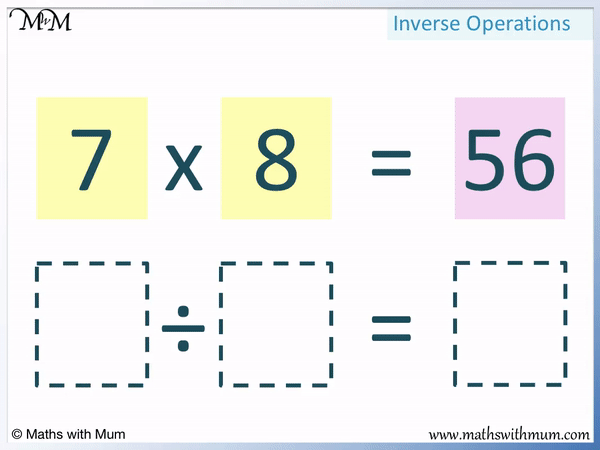The next step is to write the numbers being multiplied in the multiplication sentence after the division sign and after the equals sign in any order.

The two numbers being multiplied are 7 and 8. We will write 7 after the division sign and 8 after the equals sign.

We rearrange the multiplication 7 × 8 = 56 to the division 56 ÷ 7 = 8. The numbers involved are the same but they are written in a different order.

Whilst 56 needs to go at the start of the division, the other two numbers of 7 and 8 can be written in either order. There are two different divisions that can be written for each multiplication.

This time we will write these two numbers in a different order.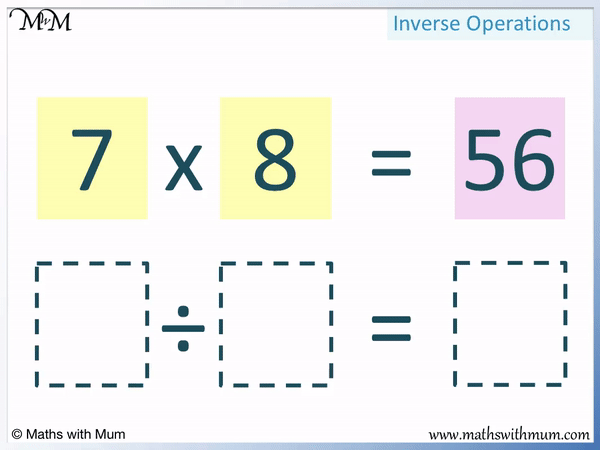We rearrange the multiplication 7 × 8 = 56 to the division 56 ÷ 8 = 7.

7 × 8 = 56 can be written as either 56 ÷ 7 = 8 or 56 ÷ 8 = 7.## How to Write Division as Multiplication

To write a division as a multiplication, follow these steps:

1. Write the number being divided as the answer at the end of the multiplication.
2. Write the remaining two numbers in the division sentence multiplied together in the multiplication sentence.
3. The two numbers in step two can be written in either order to form two different multiplication sentences.

For example, here is 18 ÷ 6 = 3

The first step is to write the number being divided, at the start of the division as the answer at the end of the multiplication.

18 is the number being divided. It will form the answer at the end of the multiplication.The next step is to write the remaining two numbers from the division multiplied together in the multiplication sentence.

The other two numbers in the division are 6 and 3.

We can write 18 ÷ 6 = 3 as 6 × 3 = 18.

We can also write 18 ÷ 6 = 3 as 3 × 6 = 18. It does not matter which order the two numbers are multiplied in.Each division sentence can be written as two different multiplication sentences.

18 ÷ 6 = 3 can be written as either 6 × 3 = 18 or 3 × 6 = 18.Now try our lesson on Division by Sharing Equally where we learn how to introduce the idea of division as sharing equally.